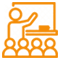Alert: You are not allowed to copy content or view source

# Singapore Math Kangaroo Contest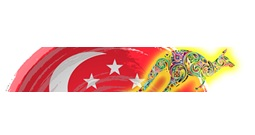## Parent Organisation:

Singapore International Math Contest Center

## About Singapore Math Kangaroo Contest:

Mathematical Kangaroo (also International Mathematical Kangaroo and Kangourou sans frontières, the original French name) is an international mathematical competition with more than 70 countries that take an active part in it.  The key competence tested by the Kangaroo is logical combination, not just pure knowledge of formulas.

The Singapore and Asian Schools Math Olympiad (SASMO) has helped many students gain more confidence in international mathematics contests and over 70 students have competed in prestigious competitions in the last 2 years and won international honours.

## Syllabus:

Math Kangaroo contest content is similar to Singapore’s Mathematics Curriculum.  Problems require creativity, logical thinking and challenge students to look at problems from a different perspective.

Pre Ecolier (Primary 1 & 2)

• simple arithmetic operations with 1 digit and 2 digit numbers
• distinguishing simple figures
• time, clock. number of days in a week
• number of months in a year

Ecolier (Primary 3 & 4)

• simple arithmetic operations with 1,2,3 and 4 digit numbers
• recognizing geometric figures.
• a magic square with a sum of 15
• time, clock. number of days in a week, number of months in a year
• addition, subtraction, multiplication, division. intersection of sets
• perimeter and area of a square, a rectangle

Benjamin (Primary 5 & 6)

• magic squares
• fractions and decimals.
• clock, a calendar
• perimeter of a polygon. area of a rectangle and a triangle
• mathematical logic.
• lines and rays on a surface
• a cube, a rectangular solid. acute, right, and obtuse angles.

Cadet Level (Secondary 1 & 2)

• operations on rational numbers
• powers of natural numbers
• angles: acute, right, and obtuse.
• equations, inequalities and systems of linear equations
• area of a rectangle, a triangle and a circle
• lines and rays on a surface
• volume and surface area of geometric figures
• supplementary angles, sum of angles in a triangle and in a quadrilateral
• mathematical logic

Junior (Secondary 3 & 4)

• operations on real numbers
• functions, polynomials, equations, inequalities.
• sequences of numbers
• elements of combinatorics
• synthetic & analytic plane geometry

Student (JC 1 & 2)

• As above

## Marking Scheme:

Primary 1 to Primary 4 (Pre-Ecolier 1,2 and Ecolier 1,2)

The test for Pre Ecolier 1, 2 and Ecolier 1, 2 is of 75 minutes duration and contains 24 questions. These are grouped equally under three levels of increasing difficulty. The student starts with 24 bonus points (i.e. no student gets a negative score). 1 point will deducted for each wrong answer and No points will be deducted for no answer. The following points are awarded:

Easy: 3 points for each correct answer.

Medium: 4 points for each correct answer.

Hard: 5 points for each correct answer.

Maximum Score: 90 points (120 points for Ecolier)

Primary 5 to JC 2 (Benjamin, Cadet, Junior, Student)

The test for Benjamin (P5 & P6), Cadet (S1 & S2), Junior (S3 & S4), and Student (JC1, JC2 & Poly) is of 75 minutes duration and contains 30 questions. These are grouped equally under three levels of increasing difficulty. The student starts with 30 bonus points (i.e. no student gets a negative score). 1 point will deducted for each wrong answer and No points will be deducted for no answer. The following points are awarded:

Easy: 3 points for each correct answer.

Medium: 4 points for each correct answer.

Hard: 5 points for each correct answer.

Maximum Score: 150 points

## Registration Process:

Contest Date: 21 March, 2018 (Wednesday)

Contest Time: set by the teacher in charge

Registration fee: \$10 per student

## Benefits, Awards & Scholarships:

Platinum Certificate (maximum score)

Gold Certificate (Top 8% scorers)

Silver Certificate (Next 12%)

Bronze Certificate (Next 20%)

Participation Certificate (Rest of 60%)

Singapore

## Explore

#### SchoolPlus Program

Yearlong program for Olympiads preparation & to build necessary skills for future.

Explore MoreExplore MoreExplore More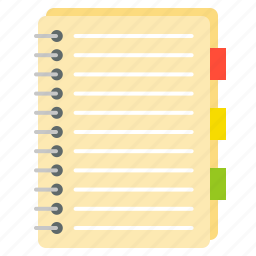Assess your performance by taking topic-wise and full length mock tests.

Explore More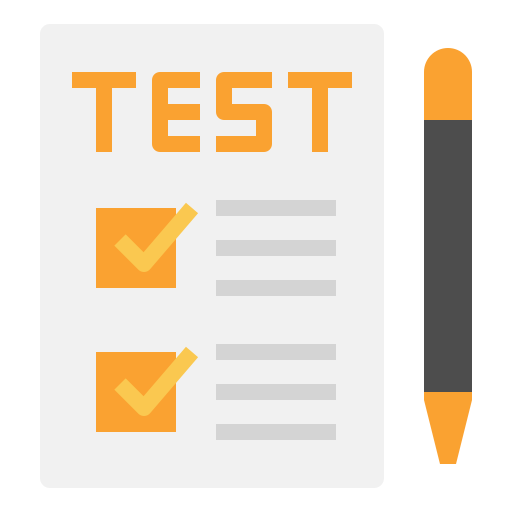Explore More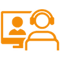Explore More